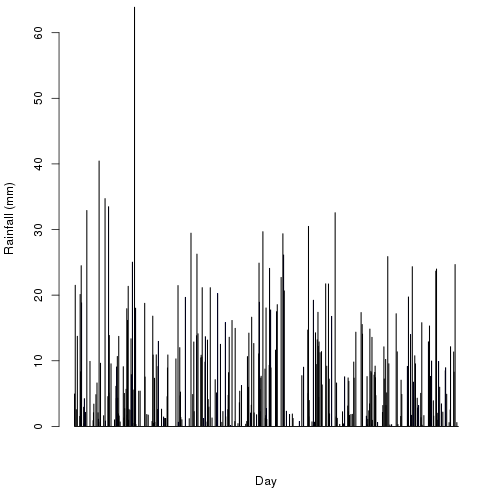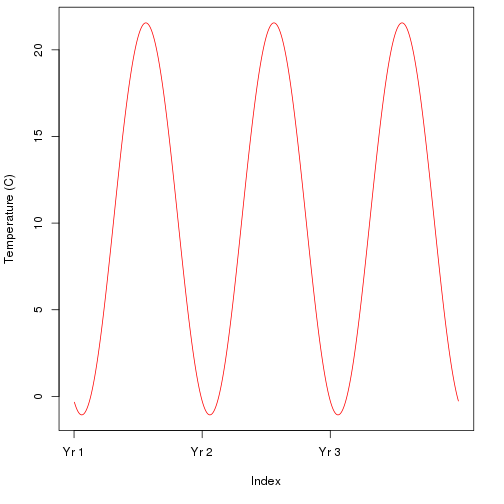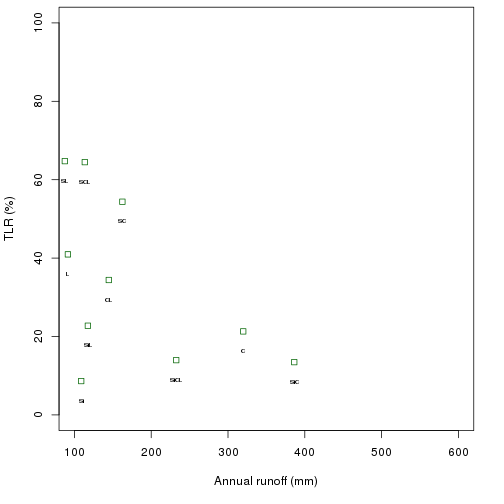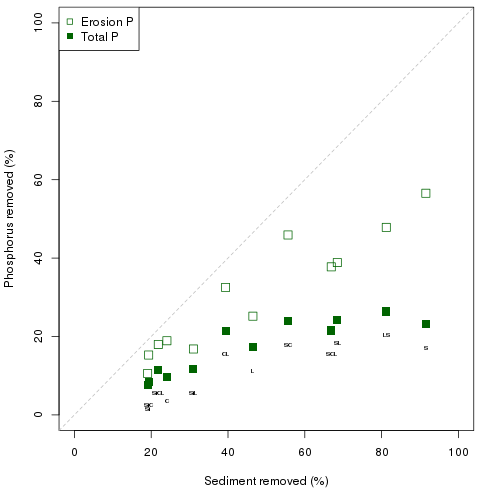The `VFS` package links a series of environmental models: rainfall, runoff, erosion, vegetated filter strip capture, and agricultural phosphorus loss. Vegetated filter strips (VFS) are a best management practice used to trap sediment from agricultural runoff along rivers and streams. The capabilities of this package are demonstrated for State College, PA. An earlier version of the `VFS` package was used by Gall et al. (2018) in their study of sediment trapping efficiency calculations.

The intent of this package is to identify agricultural areas at high risk for soil and phosphorus loss across large regions, so it is configured by default to use general parameters and return relative risks. For smaller areas, where specific model parameters are known, absolute numbers can be calculated. The models involved, particularly MUSLE (soil erosion) and APLE (phosphorus loss), are widely accepted and used extensively for conservation planning.

``````
library(VFS)
data(soildat)
data(bufferdat)

# basic required parameters
nyears <- 3
FieldArea <- 4000
FieldSlope <- 0.05
VFSslope <- 0.02
VFSwidth <- 15
soilP <- 120
soilOM <- 2
``````

## Climate simulation

The GHCN import function `read.dly` can read daily GHCN data from the GHCN FTP site, or from a local file. If you have a different source of daily weather data to use, that can be imported into R using the appropriate tools. Measured weather data can be used directly in the models, or weather data can be simulated for long periods based on parameters generated from daily weather data containing precipitation, minimum temperature, and maximum temperature by the function `wth.param`.

For this example, ten years of daily weather data is used to calculate parameters for a Markov chain rainfall simulation, and then three years of rainfall are generated. For research use, longer timespans should be used for both parameterization and for simulation, often thirty years of measured data, and 1,000 years for the simulations.

Note that leap days, if present, are removed from the data before the parameters are calculated.

``````    # State College, PA GHCN data
data("weather")
weather.param <- wth.param(weather, method="markov")

rainfallSC <- rainfall(365*nyears, weather.param)
temperatureSC <- temperature(365*nyears, weather.param)
``````## Runoff, erosion, and filter strip models

Three types of models are implemented in the `VFS` function. Runoff is calculated as a function of daily soil water balance, given crop growth, soil texture, and rainfall. Two erosion models are run automatically, a discharge-concentration (C-Q) model, and `MUSLE`. For this demonstration, the State College weather simulations is used in conjunction with each of the twelve standard soil texture classes. The output of the erosion models becomes input of the vegetated filter strip model.

``````    # bluegrass filter strip
vfsSC <- lapply(seq_len(nrow(soildat)), function(i)
VFS(nyears=nyears, thissoil=soildat[i,],
thisbuffer=subset(bufferdat, Species == "bluegrass"),
rain=rainfallSC, temperature=temperatureSC, FieldArea=FieldArea,
VFSwidth=VFSwidth, VFSslope=VFSslope, FieldSlope=FieldSlope, b=1.5))
names(vfsSC) <- soildat\$Soil

vfsSC.summary <- data.frame(t(sapply(vfsSC, summary)))
``````## Agricultural phosphorus loss

The annual runoff and MUSLE sediment loss values (before and after the filter strip) are passed to the `APLE` function, which calculates agricultural P loss through erosion, and total P loss. Each field was assumed to have the same fertilizer and manure applications.

``````    apleSC <- lapply(vfsSC, function(x)VFSAPLE(x, soilP=soilP, OM=soilOM))
names(apleSC) <- soildat\$Soil

apleSC.summary <- data.frame(t(sapply(apleSC, summary)))
``````## References

Gall, H. E., Schultz, D., Veith, T. L, Goslee, S. C., Mejia, A., Harman, C. J., Raj, C., and Patterson, P. H. 2018. The effects of disproportional load contributions on quantifying vegetated filter strip sediment trapping efficiencies. Stoch Environ Res Risk Assess 32(8): 2369–2380. \doi 10.1007/s00477-017-1505-x